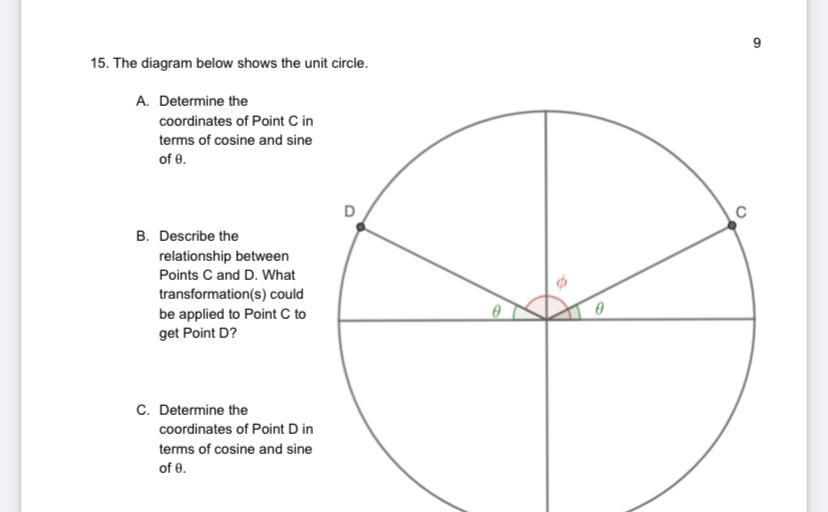Question:

# 9 15. The diagram below shows the unit circle. A. Determine the coordinates of Point Cin terms of cosine and sine of e. C B. Des9 15. The diagram below shows the unit circle. A. Determine the coordinates of Point Cin terms of cosine and sine of e. C B. Describe the relationship between Points C and D. What transformation(s) could be applied to Point C to get Point D? C. Determine the coordinates of Point Din terms of cosine and sine of e.